Search

Finding the Inverse of a Function (page 5 of 7)

Sections: Definition / Inverting a graph, Is the inverse a function?, Finding inverses, Proving inverses

• Find the inverse f(x) = (x  2) / (x + 2)where x does not equal 2.
Is the inverse a function?

 First, I recognize that f(x) is a rational function. Here's its graph: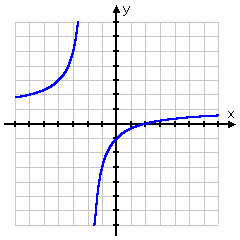The restriction on the domain comes from the fact that I can't divide by zero, so x can't be equal to 2. I usually wouldn't bother writing down the restriction, but it's helpful here because I need to know the domain and range of the inverse. Note from the picture (and recalling the concept of horizontal asymptotes) that y will never equal 1. Then the domain is "x is not equal to 2" and the range is " y is not equal to 1". For the inverse, they'll be swapped: the domain will be "x is not equal to 1" and the range will be "y is not equal to 2". Here's the algebra:

 The original function: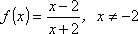I rename "f(x)" as "y":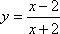Then I solve for "x =":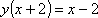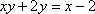I get the x-stuff on one side: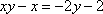Here's the trick: I factor out the x!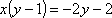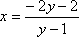Then I switch x and y: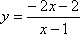And rename "y" as "f-inverse"; the domain restriction comes from the fact that this is a rational function.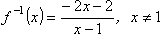Since the inverse is just a rational function, then the inverse is indeed a function.    Here's the graph: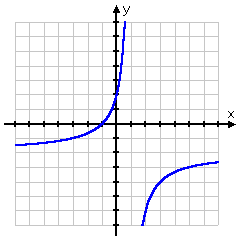Then the inverse is  y = (2x  2) / (x  1), and the inverse is also a function, with domain of all x not equal to 1 and range of all y not equal to 2.

• Find the inverse of  f(x) = x2  3x + 2,  x < 1.5

 With the domain restriction, the graph looks like this: From what I know about graphing quadratics, the vertex is at (x, y) = (1.5, 0.25), so this graph is the left-hand "half" of the parabola.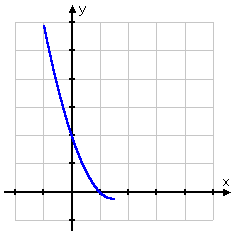This half of the parabola passes the Horizontal Line Test, so the (restricted) function is invertible. But how to solve for the inverse?  Copyright © Elizabeth Stapel 2000-2011 All Rights Reserved

 The original function: f(x) = x2  3x + 2 I rename "f(x)" as "y": y = x2  3x + 2 Now I solve for "x =" by using the Quadratic Formula: 0 = x2  3x + 2  y 0 = x2  3x + (2  y)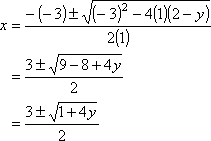Since x < 1.5, then I want the negative square root: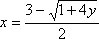Now I switch x and y: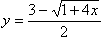And rename "y" as "f-inverse"; the domain restriction comes from the fact that this is a rational function.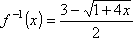Then the inverse is given by: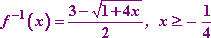<< Previous  Top  |  1 | 2 | 3 | 4 | 5 | 6 | 7  |  Return to Index  Next >>

 Cite this article as: Stapel, Elizabeth. "Finding the Inverse of a Function." Purplemath. Available from     https://www.purplemath.com/modules/invrsfcn5.htm. Accessed [Date] [Month] 2016

Study Skills Survey

Tutoring from Purplemath
Find a local math tutor

 Copyright © 2021  Elizabeth Stapel   |   About   |   Terms of Use   |   Linking   |   Site Licensing Contact Us